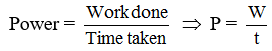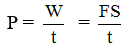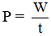# Physics

### Chapter : 3. Work and Energy

#### Power & its Unit

Definition : Power is defined as the rate of doing workIn other words, power is the work done per unit time, power is a scalar quantity.
Since W = F.S therefore= F × V = force × velocity
Unit of power : The S.I. unit of power is watt and it is the rate of doing work at 1 joule per second.
1 watt is equal to :1 kilowatt = 1 kW = 1000 W
1 Horse power = 1 H.P. = 746 W
Example : 8
A machine raises a load of 750 N through a height of 15 m in 5s. Calculate :
(i) the work done by the machine.
(ii) the power at which the machine works.
Solution. (i) Work done is given by W = F.s
Here F = 750 N; s = 15 m
∴ W = 750 × 15 = 11250 J
= 11.250 kJ
(ii) Now, power of the machine is given byHere, W = 11250 J; t = 5 s
∴ Power P = 11250J/5s = 2250 W = 2.250 kW